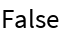# PrefixQ

Contributed by: Taliesin Beynon

Test if the first elements of a list are the same than those from another list

 ResourceFunction["PrefixQ"][list1,list2] gives True if list2 is a prefix of list1. ResourceFunction["PrefixQ"][list2] is the operator form of ResourceFunction["PrefixQ"].

## Examples

### Basic Examples

The second list starts with the same two elements of the first :

 In:=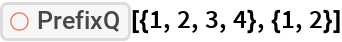Out=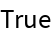A list with other elements:

 In:=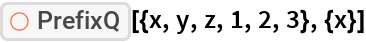Out=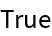A non-prefix list:

 In:=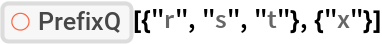Out=